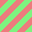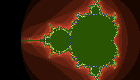acb's technical journal

# Functionally generating UIImage in Swift

When developing iOS apps, one often needs a simple UIImage, to use as a background or texture. Traditionally, the facilities for making these on the fly have been minimal to nonexistent, leaving one with two options: either (a) write code which allocates a memory buffer, fills it with pixel values, then does the CoreGraphics dance that turns it into a UIImage, or, more commonly, (b), hacks something up by hand in an image-editing program on one's computer and puts the PNG in the app bundle. The latter has the disadvantage of inflexibility (if the dimensions or colours need to change, it's back to laboriously hand-crafting a replacement image); the former, meanwhile, requires enough work to put one off, unless image generation is a central part of the app's value proposition. However, in the age of Swift, it doesn't have to be this way.

The Swift language, borrowing from functional programming, lends itself nicely to the making of labour-saving abstractions; pieces of code which implement the outline of a process, allowing the caller to provide only the code that does the specific details they want. (This has been, to a lesser extent, possible with Objective C since the addition of blocks, but Swift has further highlighted it.) In which case, it stands to reason that we should be able to do something like:

```// make an 8-by-8 black-and-white checkerboard tile
let checkerboard = UIImage(width: 8, height: 8) { (x,y) in return Float(((x/4)+(y/4))%2)  }
```

Below the cut, I present a UIImage extension which allows you to do exactly this, as well as RGB and RGBI variants.

The code is an extension of UIImage, which adds three constructors; all start with width and height parameters, and take a function which converts a (x,y) tuple (in integer pixel coordinates) into one, three or four (depending on the image type desired) Float colour values, in the range [0.0..1.0]. (The RGBA version takes an optional extra parameter, a Boolean value which, when set, indicates that the RGB values have been premultiplied with the alpha; its default value is false). The code looks as follows:

```/* Functional UIImage generation; by Andrew Bulhak http://dev.null.org/acb/  http://github.com/andrewcb/
Distributable under the Apache License */

// Helper class to assist in filling array buffers from a function
struct BufferWriter<T> {
var buffer: [T]
var index: Array<T>.Index
init(count: Int, repeatedValue: T) {
self.buffer = [T](count: count, repeatedValue: repeatedValue)
self.index = self.buffer.startIndex
}
mutating func write(value: T) {
if self.index != self.buffer.endIndex {
self.buffer[index] = value
self.index = self.index.successor()
}
}
}

extension UIImage {

/* Most of the heavy lifting takes place here */
private static func makeCGImage(width width:Int, height: Int, bytesPerPixel: Int, space:CGColorSpace?, bitmapInfo: CGBitmapInfo, @noescape writefunc: (Int, Int, inout BufferWriter<UInt8>)->())  -> CGImageRef? {
var bufferWriter = BufferWriter<UInt8>(count: width*height*bytesPerPixel, repeatedValue:0)
for i in 0..<height {
for j in 0..<width {
writefunc(j, i, &bufferWriter)
}
}

let provider = CGDataProviderCreateWithCFData(NSData(bytes: &bufferWriter.buffer, length: width*height*bytesPerPixel))

return CGImageCreate(
width, height,
8, 8*bytesPerPixel,
width*bytesPerPixel,
space,
bitmapInfo,
provider,
nil,
false,
CGColorRenderingIntent.RenderingIntentDefault)
}

/// Generate a greyscale bitmap from a (x,y)->(grey level) function
convenience init?(width: Int, height: Int, @noescape function:(Int, Int)->Float) {
guard let cgimage = (UIImage.makeCGImage(width: width, height: height, bytesPerPixel: 1, space: CGColorSpaceCreateDeviceGray(), bitmapInfo: CGBitmapInfo(rawValue: CGImageAlphaInfo.None.rawValue)) { (x, y, inout writer: BufferWriter<UInt8>)   in writer.write(UInt8(function(x,y) * 255.0))})
else { return nil }
self.init(CGImage: cgimage)
}

/// Generate a RGB bitmap from a (x,y)->(r,g,b) function
convenience init?(width: Int, height: Int, @noescape function:(Int, Int)->(Float, Float, Float)) {
guard let cgimage = (UIImage.makeCGImage(width: width, height: height, bytesPerPixel: 3, space: CGColorSpaceCreateDeviceRGB(), bitmapInfo: CGBitmapInfo(rawValue: CGImageAlphaInfo.None.rawValue)) {
(x, y, inout writer: BufferWriter<UInt8>) in
let (r,g,b) = function(x, y)
writer.write(UInt8(r * 255.0))
writer.write(UInt8(g * 255.0))
writer.write(UInt8(b * 255.0))
}) else { return nil }

self.init(CGImage: cgimage)
}

/// Generate a RGBA bitmap from a (x,y)->(r,g,b,a) function
convenience init?(width: Int, height: Int, premultiplied: Bool = false, @noescape function:(Int,Int)->(Float,Float,Float,Float)) {
let bitmapInfo = CGBitmapInfo(rawValue: (premultiplied ? CGImageAlphaInfo.PremultipliedLast : CGImageAlphaInfo.Last).rawValue)
guard let cgimage = (UIImage.makeCGImage(width: width, height: height, bytesPerPixel: 4, space: CGColorSpaceCreateDeviceRGB(), bitmapInfo: bitmapInfo) {
(x, y, inout writer: BufferWriter<UInt8>) in
let (r,g,b,a) = function(x, y)
writer.write(UInt8(r * 255.0))
writer.write(UInt8(g * 255.0))
writer.write(UInt8(b * 255.0))
writer.write(UInt8(a * 255.0))
}) else { return nil }

self.init(CGImage: cgimage)
}
}
```

With this code, the checkerboard tile example now works. And one advantage of the functional approach is that one can abstract it further, writing functions to generate images to parameters; for example, the following function which generates checkerboards of arbitrary dimensions:

```func checkerboard(width w:Int, height h:Int, rows:Int, cols:Int) -> UIImage {
return UIImage(width: w*cols, height: h*rows) { (x,y) in return Float(((x/w)+(y/h))%2) }!
}
```

Or indeed a number of other geometric shapes which lend themselves to simple functional representation; for example, tileable coloured stripes:

```func rgbStripes(width w:Int, height h:Int, stripeWidth: Int, colour1:(Float,Float,Float), colour2:(Float,Float,Float)) -> UIImage {
return UIImage(width:w, height:h) {(x, y) ->(Float,Float,Float) in
if ((x+y)/(stripeWidth*2))%2 == 1 {
return colour2
} else {
return colour1
}
}!
}

rgbStripes(width:32, height:32, stripeWidth:4, colour1:(1.0,0.5,0.5), colour2:(0.5,1.0,0.5))
```

Which gives us the following image:This isn't limited to simple examples; for example, here is an example which renders the Mandelbrot set:

```func mandelbrot(width w:Int, height h:Int, maxIterations:Int=1000) -> UIImage {
return UIImage(width:w, height:h) {(px, py) -> (Float,Float,Float) in
let x0 = ((Float(px)/Float(w))*3.5) - 2.5
let y0 = ((Float(py)/Float(h))*2.0) - 1.0
var x: Float = 0.0, y: Float = 0.0
var iteration = -1
while iteration= 4.0) { break }
(y,x) = (2*x*y + y0, x*x - y*y + x0)
iteration += 1
}
return ( (Float(iteration)*0.1)%1.0, (Float(iteration)*0.0333)%1.0, (Float(iteration)*0.01)%1.0)
}!
}

let mandel = mandelbrot(width: 140, height: 80, maxIterations:100)
```

Which produces the following:The code is also in a gist on GitHub, here.

### Want to say something? Do so here.

Post pseudonymously

To prove that you are not a bot,please enter the text in the image on the rightin the field below it.